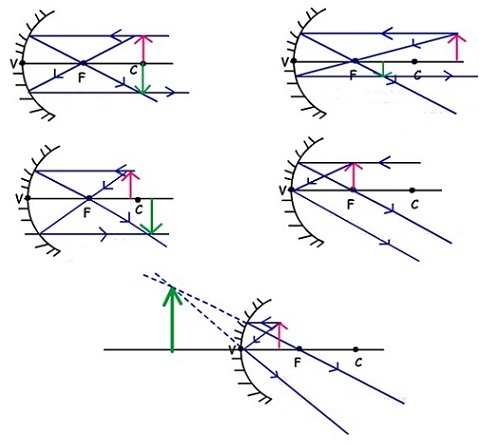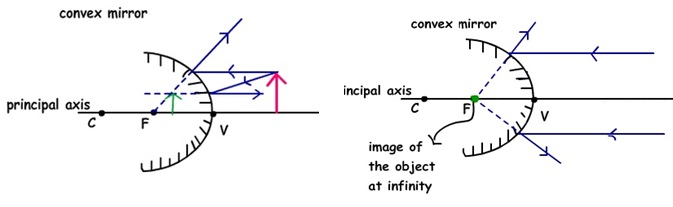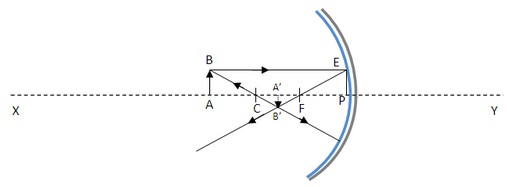#### Reflection at Curved Mirrors, Physics tutorial

Types of Curved Mirror:

We encompass two kinds of curved mirrors namely the concave and the convex mirrors. They are building up of silvering a glass surface that is a part of the sphere.

If the outside surface of the spherical portion is silvered and the internal surface of the reflecting portion, the resultant mirror is termed as a concave mirror. On the other hand, if the internal surface is silvered and the exterior surface is the reflecting part, the resultant mirror is termed as convex mirror.

Principal rays for locating images in a curved mirror:

Whenever you wish for to construct ray diagrams, you will employ the given principal rays to locate the image position of your object.

1) For a concave mirror:

• Incident rays parallel to the principal axis are reflected via the principal focus.
• Incident rays passing via centre of curvature meet up the mirror at right angle and so are reflected back all along their own paths.
• Incident rays passing via the principal focus are reflected parallel to the principal axis.

2) For a convex mirror:

Incident rays parallel to the principal axis are reflected in such a way that they appear to come from the principal focus.

Incident rays traveling in the direction of the centre of curvature meet up the mirror at right angles and therefore are reflected back all along their own paths.

Image Formed by Curved Mirrors:

The size, nature and position of the image made by a curved mirror based on the position of the object from the pole of the mirror. We can study there by the utilization of an illuminated object, a concave mirror and a screen.

To perform this, you will put the concave mirror on a stand and let light from the illuminated object to fall centrally on it by the adjustment of the height of the mirror. The mirror is a little turned to one side so as to make an image where possible on the screen. You can do this for different object positions.

If you employ a convex mirror, you will get an erect and virtual image smaller than the object position.

Images Formed by Concave Mirror:1) If the object is placed at the center of the mirror, then image is as well formed at center, real, inverted and having the similar size as object.

2) If the object is placed away from the center of the mirror, image is made between the focal point and center of the mirror. Properties of mirrors are, real, inverted and smaller in size.

3) If the object is placed between the focal point and center of the mirror, then the image is made away from the center. Features of the image are real, inverted and magnified in size.

4) If the object is placed at focal point and as you can view reflected rays goes parallel to one other. In another words, they don't intersect in any point, therefore we suppose that image is made at infinity.

5) If the object is placed between the focal point and vertex then the virtual image is formed behind the mirror. As the rays reflected from the mirror don't intersect, their extensions behind the mirror intersect and virtual image is made. Image is magnified in size and erect dissimilar the real images.

Images Formed by Convex Mirror:1) Object between infinity and pole: If the object is between infinity and the pole of a convex mirror, a diminished, virtual and erect image is made between pole and focus behind the mirror.

2) Object at infinity: If the object is at infinity, a point sized image is made at principal focus behind the convex mirror.

Distinction between Real and Virtual Images:

1) Real image can be captured in a screen whereas virtual image can't be captured in a screen.

2) Real image is for all time inverted whereas virtual image is for all time erect.

3) In real image, light rays in reality meet up to form a real image whereas in virtual image, light rays don't in reality meet.

Uses (Applications) of Curved Mirrors:

1) Object between 'F' and 'P' of a concave mirror:

At this position, the concave mirror is employed as dentist mirror, make-up mirror and showing mirror as the image formed in this position of object is enlarged, erect and virtual.

2) Object at 'f' of a concave mirror:

At this object position, the concave mirror is employed for torch. As well, parabolic concave mirrors are employed in car head-lamps and search-light. They generate a broad parallel beam of light of constant intensity if a small light source is positioned at its focus.

3) Object between 'f' and 'c':

At object position, concave mirrors are employed in several type of flood light.

4) Object at 'c':

Concave mirror is employed as projector lamp in the object position.

• Concave mirrors are as well employed as reflectors in reflecting the telescope and microscopes.
• Convex mirrors are employed as driving mirrors as they for all time provide an erect image of an object behind the driver. Moreover, they give a broader field of view than a plane mirror of the similar aperture. This makes it possible for the driver to view objects with in a large angle.

Mirror Formula and Sign Conventions:Assume that AB be an object positioned beyond C before the concave mirror with pole P and focus F. Let it make an inverted real image at A', A'B'.

Supposition: Paraxial Approximation, that is, ray AB is much close to pole P of the mirror.

From simple geometry Δ ABC ≈ Δ A'B'C

Thus,

(A'B')/AB = (A'C)/AC

Again, Δ FPE ≈ Δ A'B'C

Therefore, (A'B')/EP = A'F/FP

But EP = AB (since BE || XY)

Thus, (A'B')/AB = A'F/FP

From both the equation, we have

(A'C)/AC = A'F/FP

(CP - A'P)/(AP - CP) = (A'P - FP)/FP

Again, CP = 2FP (Radius of curvature = 2 x focal length)

Now AP = -u, A'P = -v, FP = -f, CP = 2FP = -2f

From the above equation,

=> [-2f-(-v)]/[(-u) - ( -2f)] = [(-v) - (-f)]/(-f)

On resolving, we get

1/f = (1/u) + (1/v)

Sign Convention for Spherical Mirrors:

The given sign convention is employed for measuring various distances in the ray diagrams of spherical mirrors:

1) All the distances are computed from the pole of mirror.

2) Distances evaluated in the direction of incident ray are positive and the distances measured in the direction opposite to that of the incident rays are negative.

3) Distances evaluated above the principal axis are positive and that measured beneath the principal axis are negative.

Defects of Spherical Mirrors:

We will notice spherical aberration if the aperture of a spherical mirror is big. We will as well familiar that a beam of light parallel to the axis doesn't converge to the principal focus. Such a mirror brings rays close to the principal axis to a focus further away from the pole of the mirror than the focus. Moreover, it is found that the reflected rays are tangents to a curve termed as a caustic curve. The incapability of such spherical mirror of broad aperture to bring all the parallel rays to similar focus is termed as spherical aberration. In order to ignore this phenomenon, spherical mirrors of small apertures are generally employed. As well, parabolic mirrors, where all the parallel rays are brought to a single focus are employed.

To shun the blurred images due to spherical aberration, parabolic mirrors are employed to generate parallel beams in search lights, torch lights and car head-lamps.

Tutorsglobe: A way to secure high grade in your curriculum (Online Tutoring)

Expand your confidence, grow study skills and improve your grades.

Since 2009, Tutorsglobe has proactively helped millions of students to get better grades in school, college or university and score well in competitive tests with live, one-on-one online tutoring.

Using an advanced developed tutoring system providing little or no wait time, the students are connected on-demand with a tutor at www.tutorsglobe.com. Students work one-on-one, in real-time with a tutor, communicating and studying using a virtual whiteboard technology.  Scientific and mathematical notation, symbols, geometric figures, graphing and freehand drawing can be rendered quickly and easily in the advanced whiteboard.

Free to know our price and packages for online physics tutoring. Chat with us or submit request at info@tutorsglobe.com